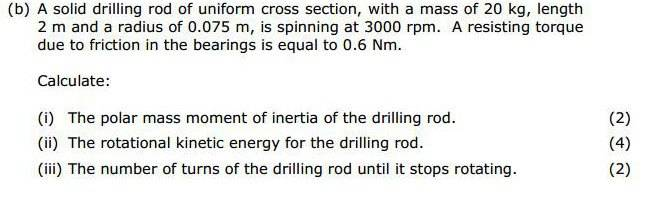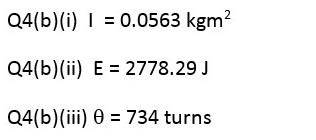# Number of Turns/Circular MotionHaving problems with (b)(iiI).

I've tried using the kinetic energy value = work done and dividing that by torque.

I've also tried used the other circular motion formulas but haven't had any success.

Attempt:

Wd = θ x T

Ek(from b ii) = Wd

θ = 2278.29/0.6
= 3797 turns

Last edited:

SteamKing
Staff Emeritus
Homework Helper
Show us what you've tried.

Show us what you've tried.

BvU
Homework Helper
I'm asking about a different question here, previous one was in regards to (b) (i), this is (b)(iii).

CWatters